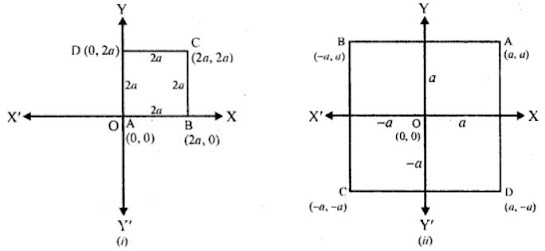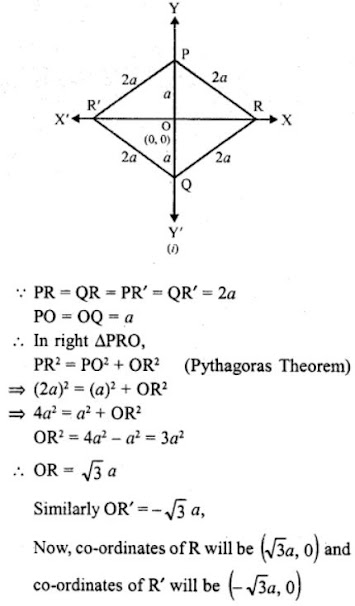# RD Sharma Solutions Chapter 14 Coordinate Geometry Exercise 14.1 Class 10 MathsChapter Name RD Sharma Chapter 14 Coordinate Geometry Book Name RD Sharma Mathematics for Class 10 Other Exercises Exercise 14.1Exercise 14.2Exercise 14.3Exercise 14.4Exercise 14.5VSAQs and MCQs Related Study NCERT Solutions for Class 10 Maths

### Exercise 14.1 Solutions

1. On which axis do the following points lie?

(i) P (5, 0)
(ii) Q (0 – 2)
(iii) R (-4, 0)
(iv) S (0, 5)

Solution

(i) P (5, 0)
Its ordinate or y-axis is 0. It lies on x-axis

(ii) Q (0 – 2)
Its abscissa or x-axis is 0. It lies on y-axis

(iii) R (-4, 0)
Its ordinate is 0 It lies on x-axis

(iv) S (0, 5)
Its abscissa is 0. It lies on y-axis

2. Let ABCD be a square of side 2a. Find the coordinates of the vertices of this square when

(i) A coincides with the origin and AB and AD are along OX and OY respectively.
(ii) The centre of the square is at the-origin and coordinate axes are parallel to the sides AB and AD respectively.

Solution

ABCD is a square whose side is 2a

(i) A coincides with origin (0, 0)
AB and AD are along OX and OY respectively
Co-ordinates of A are (0, 0), of B are (2a, 0) of C are (2a, 2a) and of D are (0, 2a)

(ii) The centre of the square is at the origin (0, 0) and co-ordinates axes are parallel to the sides AB and AD respectively.
Then the co-ordinates of A are (a, a) of B are (-a, a), of C are (-a, -a) and of D are (a, -a) as shown in the figure given below:3. The base PQ of two equilateral triangles PQR and PQR’ with side 2a lies along y- axis such that the mid-point of PQ is at the origin. Find the coordinates of the vertices R and R’ of the triangles.

Solution

∆PQR and PQR' are two equilateral triangles with side 2a each and base PQ and mid of point of PQ is O(0, 0) and PQ lies along y-axis.